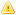Home |Sign in | EnglishHelpWLanguageWLanguage functionsStandard functionsFunctions for managing numeric valuesStatistical functionsStatistical functionsRelated examplesSee alsoStatAverageStatAverageDeviationStatCorrelationStatCovarianceStatErrorStatMaxStatMinStatStandardDeviationStatStandardDeviationPStatSumStatVarianceStatVariancePStatistical constantsStatistical functionsThe statisticsThis content has been translated automatically. Click here to view the French version.
WINDEVWEBDEVWINDEV MobileOthersStatistical functions
The statistical functions are as follows:
 StatAverage Calculates the arithmetic, geometric or harmonic mean for a series of values. StatAverageDeviation Calculates the average deviation of values in relation to their arithmetic mean. StatCorrelation Calculates the coefficient of correlation between two series of values. StatCovariance Calculates the covariance between two series of values. StatError Returns the error number for the last error caused by a Statxxx function (statistical functions). StatMax Calculates the maximum value for a series of values. StatMin Calculates the minimum value for a series of values. StatStandardDeviation Calculates the standard deviation for a series of values. StatStandardDeviationP Calculates the standard deviation for a full series of values. StatSum Calculates the sum for a series of values. StatVariance Calculates the variance for a series of values. StatVarianceP Calculates the variance for a full series of values.
Related Examples:Unit examples (WINDEV): The Stat functions [ + ] Using the main functions for statistical calculations (using matrices):- Calculate a sum of values- Calculate a mean of values- Find the minimum value in a set of values- Find the maximum value in a set of valuesUnit examples (WINDEV Mobile): The Stat functions [ + ] Using the main functions for statistical calculations (using matrices):- Calculate a sum of values- Calculate a mean of values- Find the minimum value in a set of values- Find the maximum value in a set of valuesUnit examples (WEBDEV): The Stat functions [ + ] This example presents the main functions for statistical calculations (using matrices) of WEBDEV and it allows you to:- Calculate a sum of values- Calculate an average of values- Find the minimum value in a series of values- Find the maximum value in a series of values